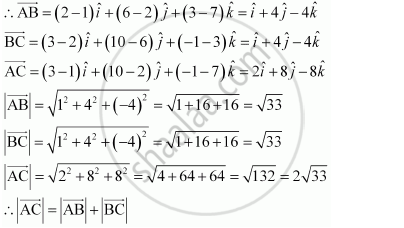Share

# Show that the Points a (1, 2, 7), B (2, 6, 3) and C (3, 10, –1) Are Collinear. - CBSE (Science) Class 12 - Mathematics

ConceptProduct of Two Vectors Projection of a Vector on a Line

#### Question

Show that the points A (1, 2, 7), B (2, 6, 3) and C (3, 10, –1) are collinear.

#### Solution

The given points are A (1, 2, 7), B (2, 6, 3), and C (3, 10, –1).Hence, the given points A, B, and C are collinear.

Is there an error in this question or solution?

#### Video TutorialsVIEW ALL 

Solution Show that the Points a (1, 2, 7), B (2, 6, 3) and C (3, 10, –1) Are Collinear. Concept: Product of Two Vectors - Projection of a Vector on a Line.
S